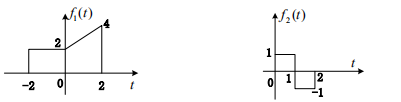# 请简要说明交互概述图在建模时的优点和缺点。并简要描述交互概述图的绘制方法。参考答案

f1(t)、f2(t)波形如图4所示，f(t)=f1(t)*f2(t)，则f(2)=()。A.1/2B.1C.3/2D.2

f1(t)、f2(t)波形如图4所示，f(t)=f1(t)*f2(t)，则f(2)=()。A.1/2

B.1

C.3/2

D.2

A.1/s+1

B.s+1/(s+1)^2+4

C.s/s^2+4

D.2/s^2+4

δ(at)，(a≠0)等于()。

A.δ(t)

B.δ’(t)

C.aδ(t)

D.1/|a|δ(t)

A.不存在

B.z/(z-1/2)+z/(z-2)

C.z/(z-1/2)-z/(z-2)

D.z/(z-2)-z/(z-1/2)1568381377

¥49.8
0.54元/天
3个月
¥39.8
1.28元/天
1个月
¥99.8
0.27元/天
1年微信付款支付宝付款